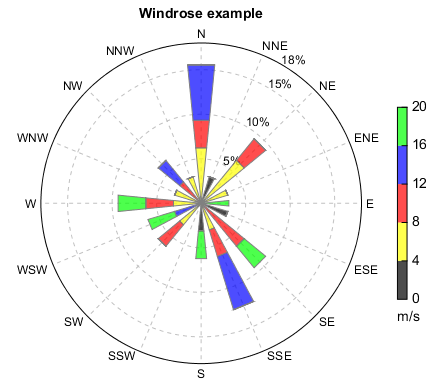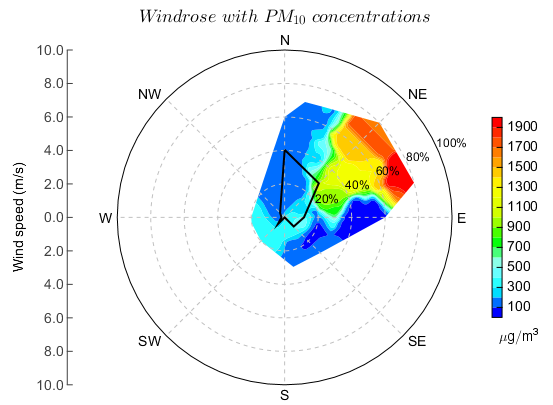# Windrose plot¶

Windrose can be plotted using windrose function with wind direction and wind speed data.

fn = r'D:\Temp\ascii\windrose.txt'
ncol = numasciicol(fn)
nrow = numasciirow(fn)
ws=a[:,0]
wd=a[:,1]

n = 16
wsbins = arange(0., 21.1, 4)
cols = makecolors(['k','y','r','b','g'], alpha=0.7)
rtickloc = [0.05,0.1,0.15,0.18]
ax, bars = windrose(wd, ws, n, wsbins, rmax=0.18, colors=cols, rtickloc=rtickloc)
colorbar(bars, shrink=0.6, vmintick=True, vmaxtick=True, xshift=10, \
label='m/s', labelloc='bottom')
title('Windrose example')In fact, windrose is plotted in a polar axes. But there are some diffenrence between windrose and polar coordinates. The polar angle theta is the counterclockwise angle from the x-axis in polar coordinates, meanwhile the polar angle theta is the colockwise angle from the y-axis in windrose coordinates. So the wind direction data have to be converted from windrose coordinates to polar coordinates for visualization purpose. The conversion processe was done in windorose function. Also you can do it in the code to plot windrose without windrose function.

The below example will plot PM10 concentration with filled contours and wind direction frequency. The following data were used.

WS WD PM10
1 335 183.2
4 350 173.4
2 0 194
1 0 175.75
3 0 203.6
3 0 161.2
3 0 142
2 0 163
2 0 175.5
1 0 208.4
1 0 205
1 0 171.2
3 170 143.6
3 135 116
3 135 110.6
2 135 93.2
5 90 98.2
4 90 91.8
6 90 83.6
4 90 88.4
2 100 81.4
1 80 77
2 0 81.8
3 0 89.4
3 0 115.8
3 0 131.2
4 0 166.4
3 0 174
3 0 170.2
7 10 152.4
6 0 184.4
7 20 203.8
4 30 212.6
8 45 627.8
6 45 1290.4
6 45 1581.25
7 80 1711.525
7 45 1841.8
6 45 2128.4
8 75 2406.8
8 45 2576.8
8 45 2035.6
7 45 1615
5 60 1286.8
4 45 1202.4
3 70 1015.2
3 80 733.8
1 90 635.6
2 225 339.2
2 270 331.4
2 260 303.2
2 225 282.6


The PM10 data was plotted in a Cartesian axes and the wind direction frequency line was plotted in a polar axes. The two axes must have same position.

def windrose2polar(a):
r = 360 - a + 90
r[r>360] = r - 360
return r

#Read data (wind speed, weed direction, pm10)
fn = r'D:\Temp\ascii\pm10.txt'
ws = table['WS']
wd = table['WD']
pm10 = table['PM10']
N = len(ws)

#Convert from windrose coordinate to polar coordinate
rwd = windrose2polar(wd)

#Calculate frequency of each wind direction bin
wdbins = linspace(0.0, pi * 2, 9)
dwdbins = degrees(wdbins)
dwdbins = windrose2polar(dwdbins)
wdN = len(wdbins) - 1
theta = ones(wdN + 1)
for i in range(wdN):
theta[i] = rwdbins[i] - pi/wdN
theta[wdN] = theta
wdhist = wdhist / N
nwdhist = wdhist.aslist()
nwdhist.append(nwdhist)
nwdhist = array(nwdhist)

#Polar coordinate to Cartesian coordinate
rwdc, wsc = pol2cart(rwd, ws)

#Get convexhull (minimum outer polygon of the wind points)
poly = topo.convexhull(rwdc, wsc)

#Get grid data
dd = 0.5
x = linspace(rwdc.min() - dd, rwdc.max() + dd, 50)
y = linspace(wsc.min() - dd, wsc.max() + dd, 50)
data = griddata((rwdc, wsc), pm10, xi=(x, y), method='idw', convexhull=False)

#---------------------------------------
#Plot figure
pos = [0.13, 0.1, 0.775, 0.775]

#Cartesian axes
ax = axes(position=pos, aspect='equal')
xaxis(visible=False)
yaxis(location='right', visible=False)
yaxis(location='left', shift = 50)
ylabel('Wind speed (m/s)')
levs = arange(100, 2000, 100)
cg = contourf(x, y, data, levs, cmap='BlAqGrYeOrRe', visible=False)
cg = cg.clip([poly])
#ss = scatter(rwdc, wsc, s=4, fill=False, edgecolor='b')
#patch(poly)
colorbar(cg, shrink=0.6, xshift=30, label=r'$\mu g/m^3$', labelloc='bottom')
maxv = 10
xlim(-maxv, maxv)
ylim(-maxv, maxv)

#Polar axes
axp = axes(position=pos, polar=True)
plot(theta, nwdhist, color='k', linewidth=2)
axp.set_rmax(1)
axp.set_rlabel_position(25.)
axp.set_rtick_locations([0.2,0.4,0.6,0.8,1])
axp.set_rticks(['2','4','6','8','10 m/s'])
axp.set_xtick_font(size=14)
axp.set_xticks(['E','NE','N','NW','W','SW','S','SE'])
title(r'$Windrose \ with \ PM_{10} \ concentrations$', fontsize=18)